# Hooke’s Law

Hooke ‘s law is used to calculate the elastic force that is produced by the deformation of a spring or other elastic materials. When an elastic body is compressed or stretched , a restoring force tends to return it to its original shape. Such force is proportional to the deformation suffered by the body, as well as its elastic constant.

Elastic force is an important application of Newton’s laws and, from there, it is possible to easily understand the behavior of Newton’s 3rd law , the law of action and reaction.

## Definition of elastic force (Hooke’s law)

The elastic force is defined from Hooke’s law. This law states that when a force is applied to a spring, it deforms, giving rise to an elastic force that has the same direction as the external force, but the opposite direction.

According to Hooke’s law, the elastic force has a magnitude equal to the force applied to the spring. Furthermore, this force is also equal to the product of the spring constant of the spring (k), measured in newtons per meter (N/m), and the spring strain (x), measured in meters.

The formula for Hooke ‘s law is as follows: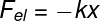In the previous figure, it is possible to perceive the presence of a negative sign , which indicates that the elastic force always has a direction opposite to the spring deformation . If the spring is being stretched, for example, the strain x will be positive, so the spring force F will be negative.

## Hooke’s Law Graph

The graph used to analyze Hooke’s law relates the magnitude of the elastic force , in N, to the spring elongation, in m. Check out an example of this graph through the following figure:

In the graph of force versus strain , it is possible to obtain the spring constant of the spring. In this case, the elastic constant represents the slope of the line, which can be obtained by dividing the force module (F) by the deformation module (x). Watch: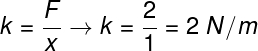## Solved exercises on Hooke’s law

Question 1 — Determine the magnitude of the deformation suffered by a spring with a spring constant of 200 N/m when subjected to a force of 50 N.

a) 10.0 m

b) 0.50 m

c) 0.25 m

d) 0.10 m

Resolution:

Let’s use Hooke’s law to calculate the deformation suffered by this spring.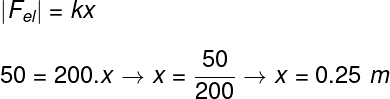Based on the calculation made in the figure above, it is possible to see that the deformation suffered by the spring is 0.25 m. Thus, the correct alternative is the letter C .

Question 2 — A spring is deformed by 10 cm (0.1 m) when compressed by a force of 200 N. Determine the spring constant of this spring.

a) 50 N/m

b) 20 N/m

c) 2000 N/m

d) 500 N/m

Resolution :

Let’s calculate the spring constant of the spring with Hooke’s law.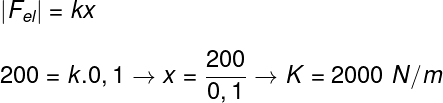Based on the result obtained, we found that the spring constant of the spring is equal to 2000 N/m, so the correct alternative is the letter D .

Question 3 — A spring with a spring constant of 500 N/m is pressed by a force of 50 N. Based on this information, calculate how much, in centimeters, the deflection of the spring should be as a result of the application of this force.

a) 100

b) 15

c) 0.1

d) 10

Resolution:

To calculate the spring deformation, it is necessary to use Hooke’s law and substitute the data provided in the exercise statement. Watch: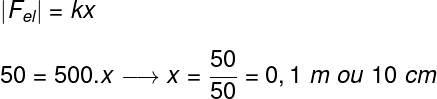Since the exercise asked us to determine the spring deformation in centimeters, after we had found the result of 0.1 m, we had to multiply it by 100, since 1 meter is 100 centimeters. Doing this, we found that the deformation was 10 cm, so the correct alternative is the letter D .